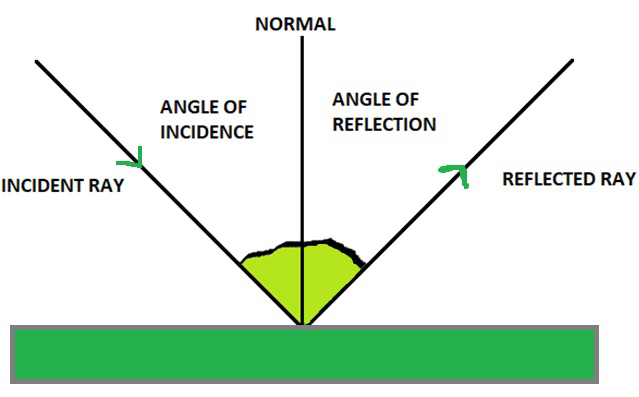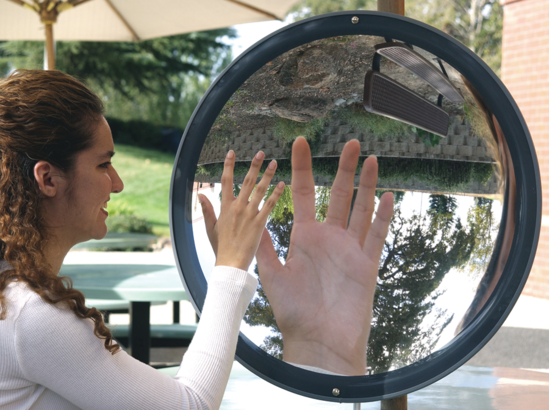Angel Pinto

laws of reflection

plane mirrors

curved mirrors

# CBSE Class 10 Science Chapter 10 Revision Notes

Light follows a straight path. When an opaque object blocks rays of light and casts a shadow on their route, this occurrence indicates that rays of light move in a straight line. When light rays strike an item, they bounce back and reach our eyes.

Table of content

# Chapter 10: Light: Reflection and Refraction Revision Notes

Definition of light

• Light is an energy type that allows us to perceive things.
• Light originates from a source and bounces off objects, which our eyes see, and our brain analyses this signal, allowing us to see.

Nature of Light

Light has the following properties:

• ray, such as a reflection
• interference and diffraction are examples of waves.
• photoelectric effect, for example

## LAWS OF REFLECTION

When light goes from one medium to another,

• it is either absorbed or reflected (absorption)
• comes back to life (reflection)
• bends or passes through (refraction)

When light strikes a flat mirror, the majority of it is reflected, while the rest is absorbed by the medium.

Light's characteristics

• Light travels at a constant speed of 2.998108m/s (roughly 3.0108m/s).

Reflection of light by other media

• A well-polished medium with no flaws on its surface will provide consistent light reflection. Consider a flat mirror.
• However, some light is absorbed by the surface even then.

Laws of ReflectionSource:

• The normal, incident ray, and reflected ray are all in the same plane.
• Angle of reflection = Angle of incidence

[∠i=∠r]

Propagation of light

• Light travels in a straight line between any two places in rectilinear propagation.
• Fermat's principle of least time may be used to justify rectilinear light propagation and the law of reflection [i=r].

Plane mirror

• A plane mirror is any flat and polished surface with nearly no flaws on its surface that reflect light.

Characteristics of images

• Real or virtual images can be upright or inverted, amplified or decreased.
• The actual convergence of light beams creates a true picture.
• The apparent convergence of divergent light beams is referred to as a virtual picture.
• If an image is generated upside down, it is referred to as inverted; otherwise, it is referred to as an upright image.
• Magnified means that the picture generated is larger than the object.
• The picture created is decreased if it is smaller than the thing.
• A planar mirror creates an image.
• A plane mirror always produces a fictitious and erect picture.
• The mirror is equidistant between the object and the image.

The Reversibility of Light Principle

• A beam of light will retrace its course if its direction is reversed owing to reflection off a surface.

Spherical Mirrors

• Consider a hollow spherical with a highly polished internal surface and a mercury-coated exterior surface that allows no light to pass through. A curved mirror, known as a spherical mirror, is created by cutting a tiny slice out of the shell.
• The focus and the radius of curvature have a relationship.
• The focal length is equal to the distance between the pole and the radius of curvature divided by two.

R/2 = F

Curved MirrorSource:

• A curved mirror is a mirror (or any polished, reflecting surface) with a curvature.
• Strictly speaking, spherical mirror is a phrase that refers to a spherical
• A spherical mirror's pole (P) is its middle.
• The spherical mirror's centre of curvature (C) was located in the centre of the sphere.
• The radius of curvature (r) is the distance between the spherical mirror and the centre of curvature. At the pole, this radius will cross the mirror (P).
• The main or primary axis is the line that passes through the pole and the centre of curvature.
• A concave mirror is a spherical mirror with an inward bulging reflecting surface.
• A convex mirror is a spherical mirror with a bulging reflecting surface.
• Take a concave mirror to focus (F). At a location halfway between the pole and the centre of curvature, all rays parallel to the primary axis converge. The focal point or focus is the name given to this place.
• Focal length is the distance between the pole and the point of focus.

Rules of ray diagram for representation of images formed

• When a ray passes through the centre of curvature, it collides with the concave spherical mirror, retracing its steps.
• The focal point or focus is where rays parallel to the primary axis pass through.

Image formation by spherical mirrors

• The image created may be discovered using the ray diagrams for the special two rays for objects in various locations.
• The table that follows is for a concave mirror.
• Based on the picture created, a spherical mirror is used.
• Concave and convex mirrors are utilised in a variety of ways on a daily basis.
• Vehicle rearview mirrors, lighting, and solar cookers are all examples.

## MAGNIFICATION AND MIRROR FORMULA

Sign convention for ray diagram

• Distances measured in the direction of positive x and y axes (coordinate system) are positive, whereas distances measured in the direction of negative x and y axes are negative.
• Keep in mind that the pole is the beginning (P).
• The height of the item is usually considered as positive if it is higher than the primary axis, and the height of the picture is usually treated as negative if it is lower than the principle axis.

Magnification and the Mirror Formula

• 1/f = 1/v + 1/u where 'u' is the object distance, 'v' is the image distance, and 'f' is the spherical mirror's focal length, which is determined by triangle similarity.
• A spherical mirror's magnification is equal to the height of the image divided by the height of the item.
• The letter 'm' is commonly used to symbolise it.
• The magnification formula m = h'/h = – (v/u) can be used to determine the size of a picture. It is a genuine picture if m is -ve, and it is a virtual image if m is +ve.

## THE REFRACTIVE INDEX AND REFRACTION THROUGH THE SLAB

Refraction

• The quickest road does not have to be the shortest.
• Because light is constantly in a hurry, it bends as it passes through a new medium because it is still on the shortest path.
• Refraction is the phenomenon of light bending in a different medium.

The Refraction Laws

• At the point of incidence, the incident ray, refracted ray, and normal to the interface of two transparent media all lie in the same plane.
• For a given colour of light and a given set of media, the ratio of the sine of the angle of incidence to the sine of the angle of refraction is constant.
• Snell's law of refraction is another name for this law.

Refractive Indexes, Absolute and Relative

• The relative refractive index is the refractive index of one media in relation to another medium.
• It's called an absolute refractive index when measured in terms of vacuum.

Refraction through a rectangular glass slab

• When light strikes a rectangular glass slab, it refracts laterally and emerges parallel to the incident beam.
• It transitions from a rarer to a denser media, then back to a rarer medium.

Refraction at a planar surface

• When travelling from a rarer to a denser medium at the surface of the two media, light bends towards the normal, according to Snell's Law.
• When light moves from a denser to a rarer medium, it bends away from the normal at the point where the two media meet.

Index of Refraction

• The refractive index describes how much light bends when it moves from one material to another.
• This is determined by the speed ratio of the two mediums.
• The bigger the ratio, the more bending there will be.
• It's also the constant ratio of the sine of the angle of incidence to the sine of the angle of refraction for each given set of media.
• It's identified by:

n = sini/sinr = light speed in medium 1/light speed in medium2.

Total internal reflection

• When light passes from a denser medium and into a rarer medium, it bends away from the regular path.
• The critical angle is the angle at which the incident ray causes the refracted ray to go parallel to the surface of the two media.
• When the incidence angle is larger than the critical angle, the light reflects rather than refracts inside the denser material.
• Total Internal Reflection is the name for this occurrence.
• Mirages and optical fibres, for example.

Lenses with a Spherical Shape

• At curved surfaces, refraction occurs.
• The principles of refraction still apply when light strikes a curved surface and travels through it.
• Consider the case of lenses.

Spherical lenses

• Spherical lenses are made by glueing together two spherical transparent surfaces.
• Convex lenses are spherical lenses made by joining two spherical surfaces that bulge outward, whereas concave lenses are spherical lenses formed by binding two spherical surfaces that are curled inward.
• Sophisticated words for spherical lenses.
• The Optical Centre (P) of a spherical lens is the halfway or symmetric centre of the lens. The pole is another name for it.
• The line that passes through the optical centre and the centre of curvature is known as the principal axis.
• A ray that is near to the major axis while simultaneously being parallel to it is known as a paraxial ray.
• The spheres that the spherical lens was a part of have their centres of curvature (C). A spherical lens has two curvature centres.
• The focus (F) of a lens is the point on the axis where parallel rays of light converge or appear to diverge after refraction.
• Focal length is the distance between the optical centre and the point of focus.
• Diverging lens: Concave lens
• Converging lens: Convex lens

Rules of ray diagram for representation of images formed

• A beam of light that passes/appears to pass through the focus is parallel to the primary axis.
• A beam that passes through the optical centre has no deviation.
• Image formation by spherical lenses.

Lens formula and magnification

• The connection between the object-distance (u), image-distance (v), and focal length (f) of a spherical lens is given by the lens formula: 1/v = 1/u = 1/f.

Uses of spherical lens

• Spectacles, binoculars, magnifying lenses, and telescopes are examples of visual aids.

Power of a Lens

• The reciprocal of a lens' focal length, i.e. 1/f, is its power (in metre).
• The dioptre is the SI unit of lens power (D).

Source: